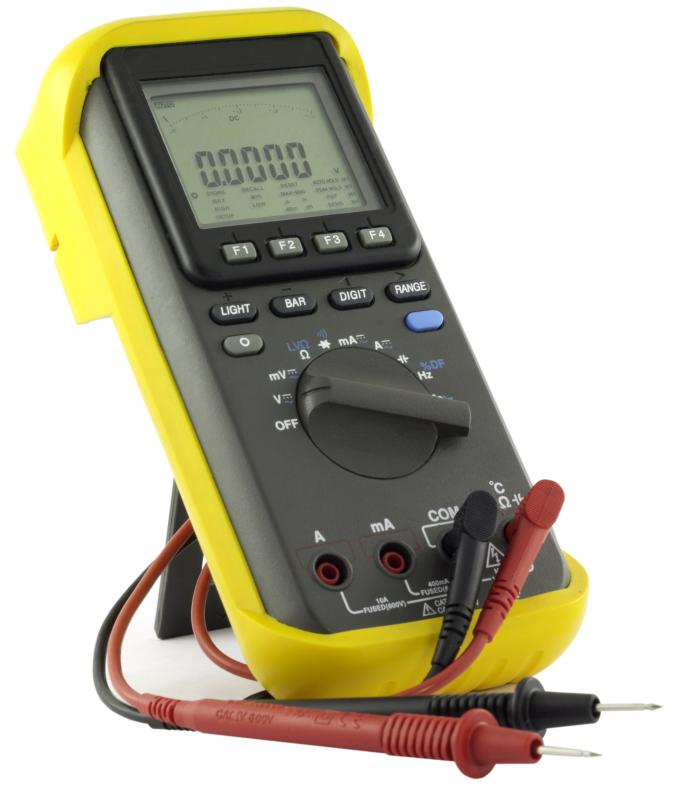# What is a Megohm? (with pictures)

J. James
J. JamesA multimeter, which can be used to measure ohms.

A megohm is a unit of measurement that describes electrical impedance. Meg- is a slightly shortened prefix for mega, which is 1x106; -ohm is the basic unit, which is represented by the Greek letter omega (Ω). A megohm, therefore, is an impedance measurement that represents one million ohms. An ohm relates a system’s resistance to passing one ampere of current between two points of a conducting medium, such as a copper wire, that is held at a constant one-volt potential. This is the basis for Ohm’s Law, named for the German physicist Georg Simon Ohm who first developed this relationship between the most basic elements of electricity in 1827.Carbon resistors apply electrical resistance of known value to circuits.

The ohm is the most widely accepted unit of measurement for electrical impedance by the International System of Units (SI). Electrical impedance is also commonly referred to as resistance, though this actually is only applicable in the case of direct current (DC). Ohm’s Law can be represented by the equation V = IR — V equals voltage that is measured in volts, I equals current that is measured in amperes, and R equals resistance of the conductor.Empirical observations made by German physicist Georg Ohm provided the basis for understanding electrical circuits.

When Ohm developed what would later be known as Ohm’s Law, he was scoffed at by colleagues and dismissed in ridicule. It would take six years to gain acknowledgment for his discovery, though he had proven that the amount of current passed through an object was directly proportional to the voltage across the material at a fixed temperature. This empirical observation performed by Ohm in 1827 provided the basis for understanding electrical circuits.

The unit of proportionality in the relationship between voltage and current is the one now bearing Ohm's name. In highly resistive circuits, the ohm is often substituted with the megohm when single ohms are not an applicable scale. Consequently, a megohm test is often used to discover the condition of a system’s insulation, also called an insulation resistance test, where the goal is to maintain a highly resistive path, such as in a refrigeration compressor.

## You might also Like## Discussion Comments

anon962194

Actually, when considering DC currents, it is "electrical resistance" - not impedance. The term "impedance" is more aptly used in the industry (and as accepted terminology) where Alternating Currents are concerned.

This is because an AC impedance has terms that are resistive as well as inductive and capacitive. An impedance value (or measurement) results from the interacting relationships of a complex (vector) conjugate of: xL, xC, xR.

Forgot password?
###### Register:
•A multimeter, which can be used to measure ohms.
•Carbon resistors apply electrical resistance of known value to circuits.
•Empirical observations made by German physicist Georg Ohm provided the basis for understanding electrical circuits.